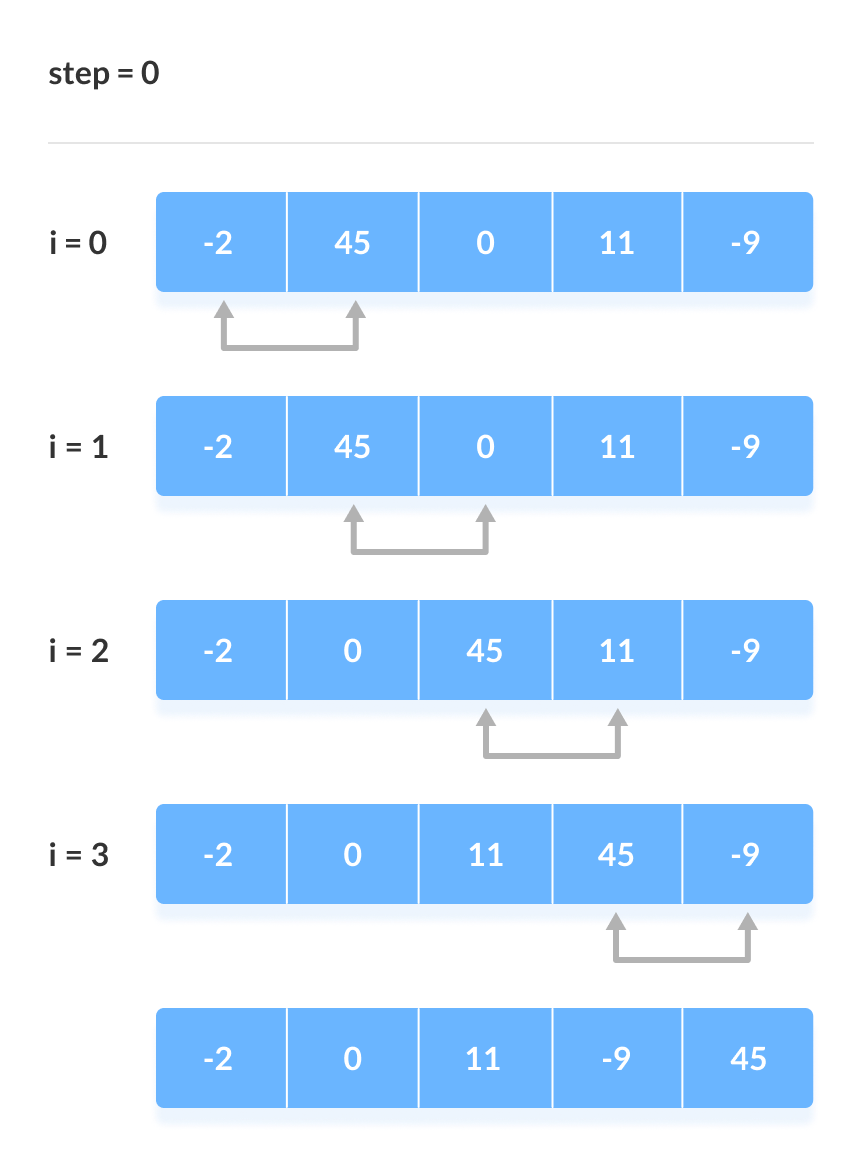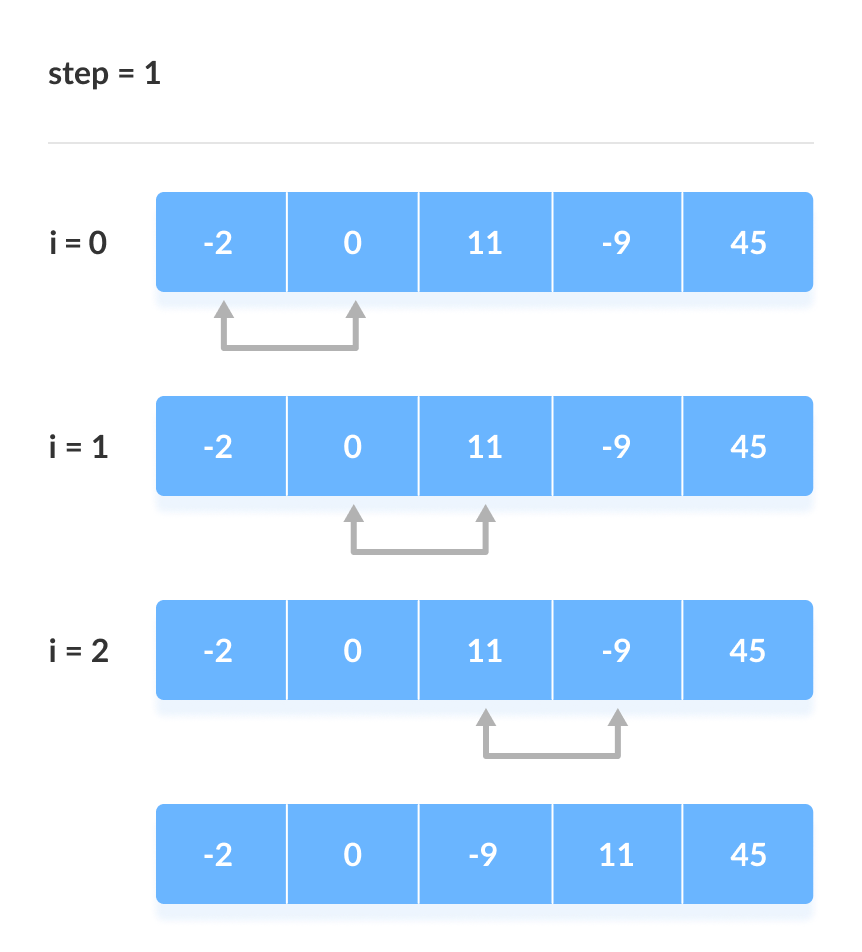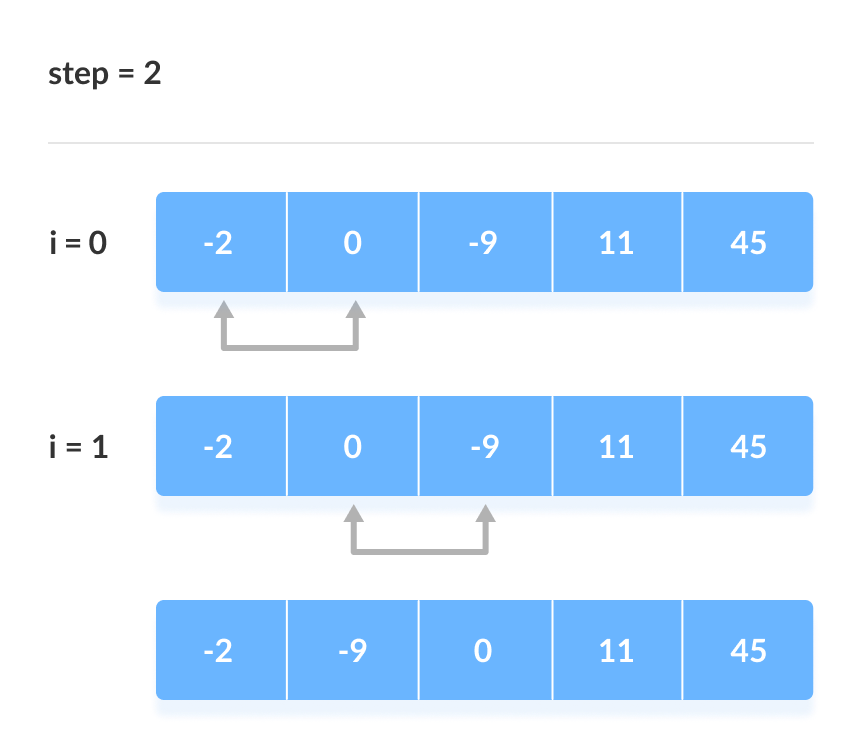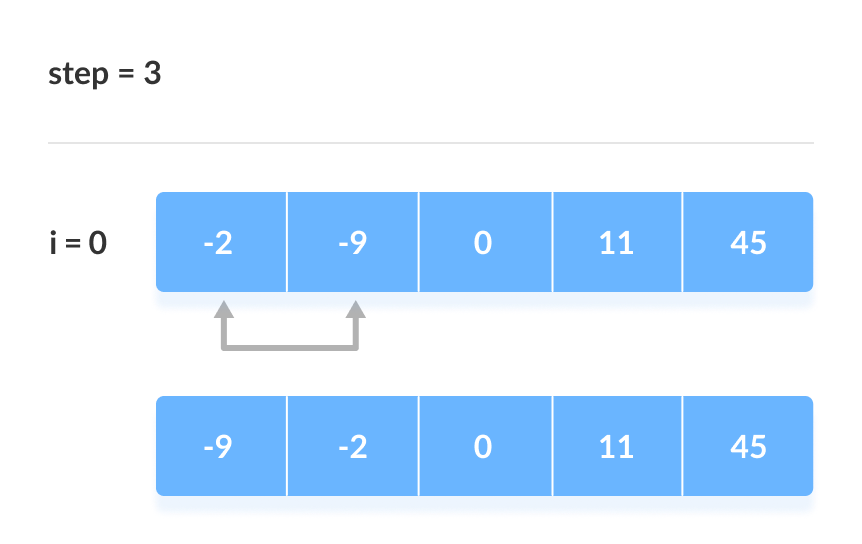Bubble Sort Algorithm

In this tutorial, you will learn how bubble sort works. Also, you will find working examples of bubble sort in C, C++, Java and Python.

Bubble sort is an algorithm that compares the adjacent elements and swaps their positions if they are not in the intended order. The order can be ascending or descending.

Pseudocode

``````bubbleSort(array)
for step <- 0 to size-1
for i <- size-step-1
if array[i] > array[i+1]
swap array[i] and array[i+1]
end if
end for
end for
end bubbleSort
``````

How Bubble Sort Works?

Let us analyze the bubble sort algorithm for sorting in ascending order.

1. When `step=0` (ie. the first pass of the first loop) and `i=0` (ie. the first pass of the second loop), the first two elements are compared.
2. When `step=0` and `i=1`, the 2nd and 3rd elements are compared.
1. If the 2nd element is greater than the 3rd then, the elements are swapped.
2. Else, swapping is not done.
3. When `step=0` and `i=2`, ………………………
4. The process continues till `step=3` and `i=0`.Here, the value of step goes till the second last value of size (ie. `size-1`) because the array is already sorted at the second last value of n. There is no need for traversing the loop at `step=size`.

Another important thing to take into account is that the value of i goes till `(size-step-1)).` To understand this, let us take a look at the illustration above.

At the end of `step=0`, `i=(size-step-1)=5-0-1=4`. For 5 elements, we can make 4 comparisons so the value of i is 4.

At the end of `step=1`, `i =(size-step-1)=5-1-1=3`. The last position is already filled by the largest element (ie. `45`) in `step=0`. There is no need for comparing the last element and the second last element at `step=1`. Thus, the value of `i=3`.

Bubble Sort in C

``````#include <stdio.h>

void bubbleSort(int array[], int size){
for(int step=0; step<size-1; ++step){
for(int i=0; i<size-step-1; ++i){
// To sort in descending order, change > to <.
if (array[i]>array[i+1]){
int temp=array[i];
array[i]=array[i+1];
array[i+1]=temp;
}
}
}
}
void printArray(int array[], int size){
for(int i=0; i<size; ++i){
printf("%d  ", array[i]);
}
printf("\n");
}
int main(){
int data[] = {-2, 45, 0, 11, -9};
int size = sizeof(data)/sizeof(data);
bubbleSort(data, size);
printf("Sorted Array in Ascending Order:\n");
printArray(data, size);
}
``````

Bubble Sort in C++

``````#include <iostream>
using namespace std;

void bubbleSort(int array[], int size){
for(int step=0; step<size-1; ++step){
for(int i=0; i<size-step-1; ++i){
// To sort in descending order, change > to <.
if(array[i]>array[i+1]){
int temp=array[i];
array[i]=array[i+1];
array[i+1]=temp;
}
}
}
}
void printArray(int array[], int size){
for(int i=0; i<size; ++i){
cout<<"  "<<array[i];
}
cout<<"\n";
}
int main(){
int data[] = {-2, 45, 0, 11, -9};
int size = sizeof(data)/sizeof(data);
bubbleSort(data, size);
cout<<"Sorted Array in Ascending Order:\n";
printArray(data, size);
}``````

Bubble Sort in Python` `

``````def bubbleSort(array):
for i in range(len(array)):
for j in range(0, len(array)-i-1):
# To sort in descending order, change > to <.
if array[j] > array[j+1]:
array[j], array[j+1] = array[j+1], array[j]

data = [-2, 45, 0, 11, -9]
bubbleSort(data)
print("Sorted Array in Ascending Order:")
print(data)``````

Bubble Sort in Java` `

``````class BubbleSort{
void bubbleSort(int array[]){
int size = array.length;
for (int i = 0; i < size-1; i++){
for (int j = 0; j < size-i-1; j++){
// To sort in descending order, change > to <.
if (array[j] > array[j+1]){
int temp = array[j];
array[j] = array[j+1];
array[j+1] = temp;
}
}
}
}
void printArray(int array[]){
int size = array.length;
for(int i=0; i<size; ++i)
System.out.print(array[i]+" ");
System.out.println();
}
public static void main(String args[]){
int[] data = {-2, 45, 0, 11, -9};
BubbleSort bs = new BubbleSort();
bs.bubbleSort(data);
System.out.println("Sorted Array in Ascending Order:");
bs.printArray(data);
}
}
``````

Bubble Sort in Descending Order

To sort in descending order, `>` is changed to `<` on the comparison line of the code ie. if condition inside bubbleSort().

If the first element is smaller than the second then they are swapped else, they are not swapped. And the process goes on for remaining comparisons.

Optimized Bubble Sort

In the above code, all the comparisons are made even if the array is already sorted at some point. It increases the execution time.

The code can be optimized by introducing an extra variable swapped. After every pass, if there is no swapping taking place then, there is no need for performing further loops. Variable swapped is false if there is no swapping. Thus, we can prevent further iterations.

In the algorithm, step 2 is revised as below:

``````bubbleSort(array)
for each position step in the array
swapped <- false
for each position i in the array
if array[i] > array[i+1]
swap(array[i], array[i+1])
swapped <- true
end if
end for
until not swapped
end for
end bubbleSort
``````

Optimized Bubble Sort in C` `

``````#include <stdio.h>

void bubbleSort(int arrayay[], int size){
for(int step=0; step<size-1; ++step){
int swapped = 0;
for(int i=0; i<size-step-1; ++i){
// To sort in descending order, change > to <.
if(arrayay[i]>arrayay[i+1]){
int temp=arrayay[i];
arrayay[i]=arrayay[i+1];
arrayay[i+1]=temp;
swapped = 1;
}
}
// If "swapped" is unchanged, the array is already sorted.
if (swapped == 0)
break;
}
}
void printarrayay(int arrayay[], int size){
for(int i=0; i<size; ++i){
printf("%d  ", arrayay[i]);
}
printf("\n");
}
int main(){
int data[] = {-2, 45, 0, 11, -9};
int size = sizeof(data)/sizeof(data);
bubbleSort(data, size);
printf("Sorted Array in Ascending Order:\n");
printarrayay(data, size);
}``````

Optimized Bubble Sort in C++` `

``````#include <iostream>
using namespace std;

void bubbleSort(int array[], int size){
for(int step=0; step<size-1; ++step){
int swapped=0;
for(int i=0; i<size-step-1; ++i){
// To sort in descending order, change > to <.
if(array[i]>array[i+1]){
int temp=array[i];
array[i]=array[i+1];
array[i+1]=temp;
swapped = 1;
}
}
// If "swapped" is unchanged, the array is already sorted.
if (swapped == 0)
break;
}
}
void printArray(int array[], int size){
for(int i=0; i<size; ++i){
cout<<"  "<<array[i];
}
cout<<"\n";
}
int main(){
int data[] = {-2, 45, 0, 11, -9};
int size = sizeof(data)/sizeof(data);
bubbleSort(data, size);
cout<<"Sorted Array in Ascending Order:\n";
printArray(data, size);
}``````

Optimized Bubble Sort in Python

``````def bubbleSort(array):
for i in range(len(array)):
swapped=True
for j in range(0, len(array)-i-1):
# To sort in descending order, change > to <.
if array[j] > array[j+1]:
array[j], array[j+1] = array[j+1], array[j]
swapped = False
if swapped=True:
break

data = [-2, 45, 0, 11, -9]
bubbleSort(data)
print("Sorted Array in Ascending Order:")
print(data)``````

Optimized Bubble Sort in Java` `

``````class BubbleSort{
void bubbleSort(int array[]){
int size = array.length;
for (int i = 0; i < size-1; i++){
boolean swapped = true;
for (int j = 0; j < size-i-1; j++){
// To sort in descending order, change > to <.
if (array[j] > array[j+1]){
int temp = array[j];
array[j] = array[j+1];
array[j+1] = temp;
swapped = false;
}
}
if (swapped == true)
break;
}
}
void printArray(int array[]){
int size = array.length;
for(int i=0; i<size; ++i)
System.out.print(array[i]+" ");
System.out.println();
}
public static void main(String args[]){
int[] data = {-2, 45, 0, 11, -9};
BubbleSort bs = new BubbleSort();
bs.bubbleSort(data);
System.out.println("Sorted Array in Ascending Order:");
bs.printArray(data);
}
}``````

Complexity

Bubble Sort is one of the simplest sorting algorithms. Two loops are implemented in the algorithm.

Pass Number of Comparisons
1st (n-1)
2nd (n-2)
3rd (n-3)
....... ......
last 1

Number of comparisons: `(n-1) + (n-2) + (n-3) +.....+ 1 = n(n-1)/2` nearly equals to `n2`

Complexity = O(n2)

Also, we can analyze the complexity by simply observing the number of loops. There are 2 loops so the complexity is n*n = n2

Time Complexities

• Worst Case Complexity: O(n2)
If we want to sort in ascending order and the array is in descending order then, the worst case occurs.
• Best Case Complexity: O(n)
If the array is already sorted, then there is no need for sorting.
• Average Case Complexity: O(n2)
It occurs when the elements of the array are in jumbled order (neither ascending nor descending).

Space Complexity

Space complexity is O(1) because an extra variable temp is used.
If the variable is swapped then it adds to space complexity thus, making it O(2).

Bubble Sort Applications

Bubble sort is used in the following cases where

1. the complexity of the code does not matter
2. a short code is demanded